Functions for Trigonometry: Homeschool Curriculum Chapter Exam

Exam Instructions:

Choose your answers to the questions and click 'Next' to see the next set of questions. You can skip questions if you would like and come back to them later with the yellow "Go To First Skipped Question" button. When you have completed the practice exam, a green submit button will appear. Click it to see your results. Good luck!

Answered 0 of 30

Page 1

Question 1 1. If the graph of f(x) is shown below, which choice represents the graph of f(x + 1) + 2?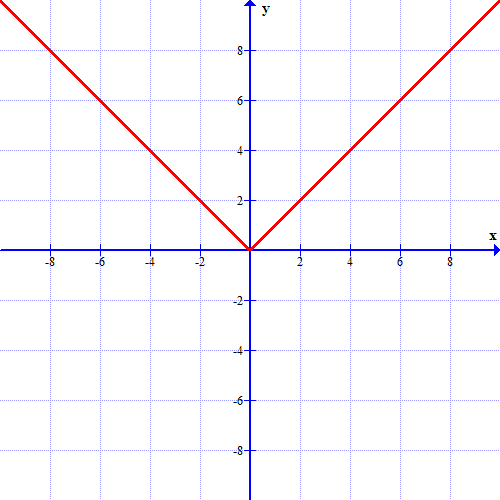Question 2 2. What is the domain of this function?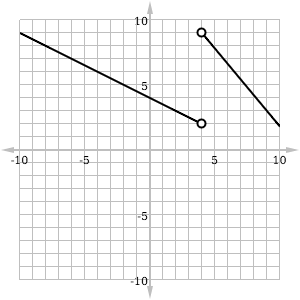Question 4 4. What is the domain of this function?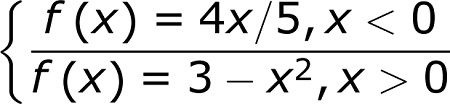Question 5 5. Which equation matches the following graph?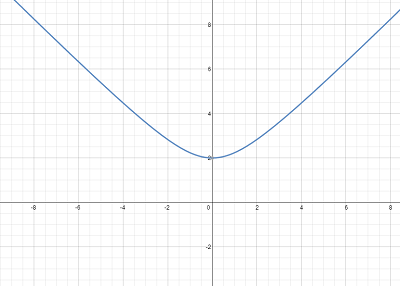Page 2

Question 6 6. In the figure shown below, the graph of the yellow function is a translation of the graph of the blue function. If the expression for the blue function is y(x) = b(x), which of the following is the equation of the yellow function?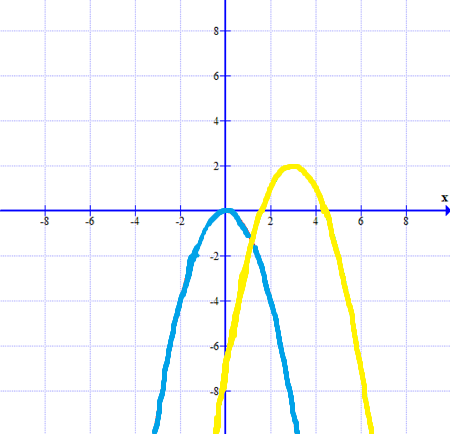Question 7 7. What is the domain of the function below?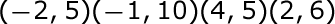Question 9 9. Which equation matches the following graph?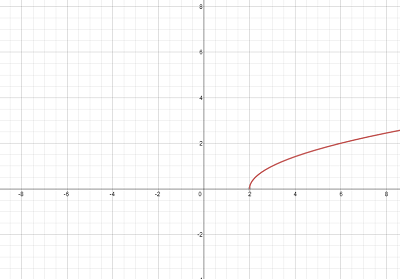Page 3

Question 14 14. What is the range of the function represented by the graph below?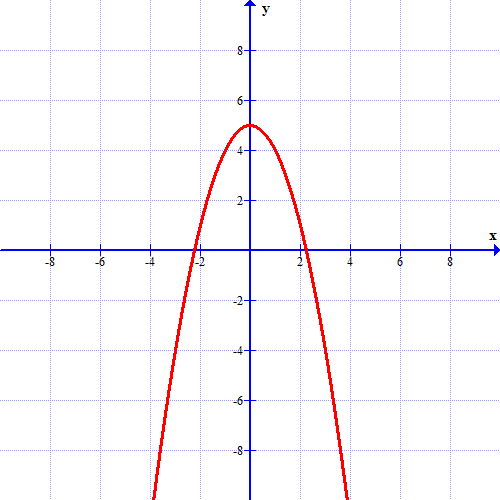Question 15 15. What is the domain of this function?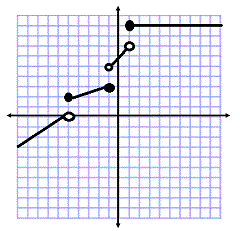Page 4

Question 18 18. Choose the answer that best describes the function of this graph.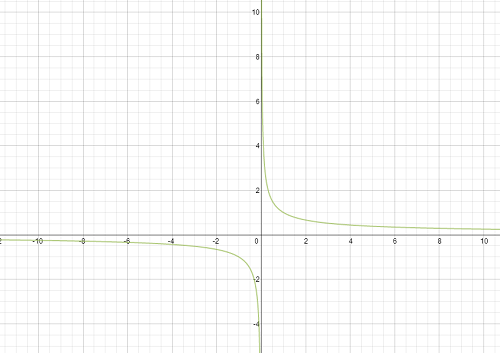Question 19 19. Which piecewise function is represented by this graph?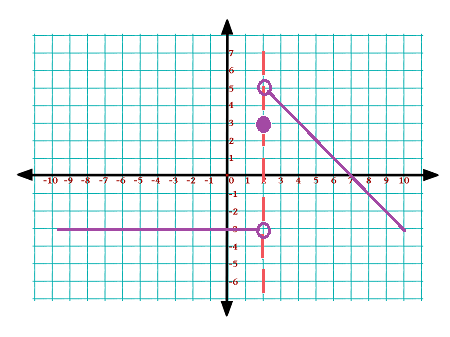Page 6

Question 28 28. Choose the answer that best describes the function of this graph.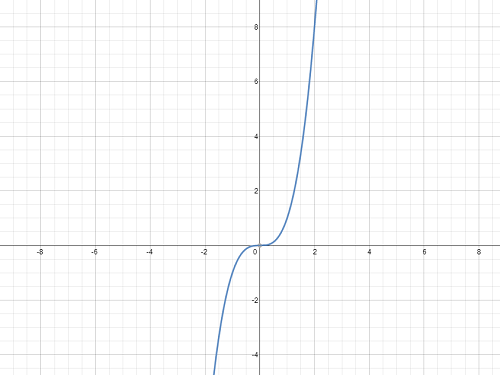Question 30 30. Which equation matches the graph?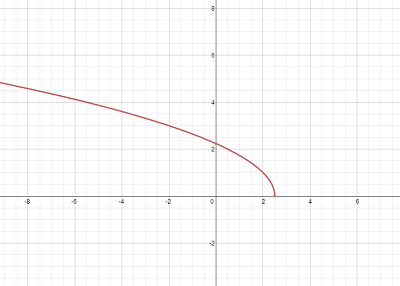Functions for Trigonometry: Homeschool Curriculum Chapter Exam Instructions

Choose your answers to the questions and click 'Next' to see the next set of questions. You can skip questions if you would like and come back to them later with the yellow "Go To First Skipped Question" button. When you have completed the practice exam, a green submit button will appear. Click it to see your results. Good luck!

Support